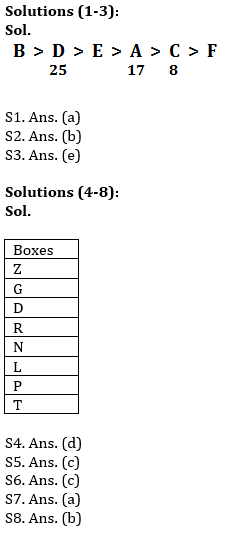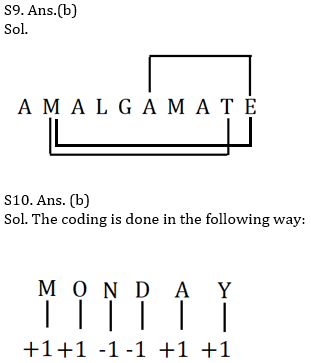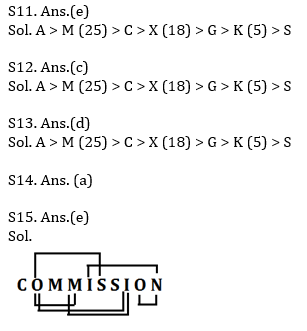Latest Banking jobs   »   Reasoning Ability Quiz For IBPS Clerk...

# Reasoning Ability Quiz For IBPS Clerk Prelims 2021- 10th August

Directions (1-3): Study the following information carefully and answer the questions given below:

Q1. What may be the possible number of books read by E?
(a) 18
(b) 26
(c) 15
(d) 12
(e) 10

Q2. How many persons read more books than E?
(a) None
(b) Two
(c) Four
(d) Five
(e) Three

Q3. Find the sum of number of books read by A and D?
(a) 33
(b) 40
(c) 38
(d) 44
(e) 42

Directions (4-8): Study the following information carefully and answer the questions given below:
Eight boxes are kept one above the other. More than five boxes are kept above box P. Box D is kept immediately above box R and immediately below box G. Two boxes are kept between box R and box Z. Box N is kept below Z and above box L. Box T is kept below box L but not immediate below. At least four boxes are kept below box R.

Q4. Which of the following is not true?
(a)Box P is kept above box T
(b)Box T is kept at the bottommost position
(c)There are two boxes kept below box L
(d) Box R is kept immediately above box L
(e)All are incorrect

Q5. Number of boxes kept below box G is same as number of boxes kept above_______?
(a)N
(b)L
(c)P
(d)R
(e)None of these

Q6. How many boxes are kept above box Z?
(a)Two
(b)Three
(c)None
(d)Five
(e)Four

Q7. Which box is kept exactly between box N and box P?
(a)L
(b)T
(c)Z
(d)R
(e)None of these

Q8. If box P is related to box L, box N is related to box R, then in the same manner box G is related to _________?
(a)D
(b)Z
(c)R
(d)T
(e)None of these

Q9. How many pairs of letters are there in the word ‘AMALGAMATE’, each of which have as many letters between them in the word as they have between them in the English alphabet (From both forward and backward directions)?
(a) one
(b) three
(c) two
(d) four
(e) None

Q10.In a certain code “MONDAY” is coded as “NPMCBZ” and “SUNDAY” is coded as “TVMCBZ”, then what will be the code for the word “FRIDAY”?
(a)ESHCBZ
(b)GSHCBZ
(c)FSHDBZ
(d)ESICBZ
(e)EQHCBZ

Directions (11-13): Read the following information carefully and answer the given questions:
Seven people A, C, G, K, S, M and X are having different ages. K is elder to S but younger to G. The difference between the ages of 2nd eldest and 4th youngest is 7 years. only two persons are elder to C. G is younger to M who is not the eldest. Age of K is not more than 5 years. X is elder to G but younger to C. Age of 2nd eldest person is the square of the number which is the age of K. Age difference between X and third youngest person is more than 10 years.

Q11. Who among the following is the 4th youngest?
(a) A
(b) K
(c) C
(d) S
(e) X

Q12. What is the age of M?
(a) 36
(b) 64
(c) 25
(d) 30
(e) Can’t be determined

Q13. What could be the possible age of C?
(a) 28
(b) 30
(c) 15
(d) 22
(e) None of the above

Q14. What should be placed in place of question mark(?) in equation E ≤ Z ? W ? C ? D to make Z > D always follow.
(a) =, >, ≥
(b) =, <, ≥
(c) =, >, ≤
(d) =, ≥, ≥
(e) None of these

Q15. How many pairs of letters are there in the word ‘COMMISSION’, each of which have as many letters between them (both forward and backward direction) in the word as they have between then in the English alphabet?
(a)One
(b)Three
(c) Two
(d)Four
(e) More than five

Solutions